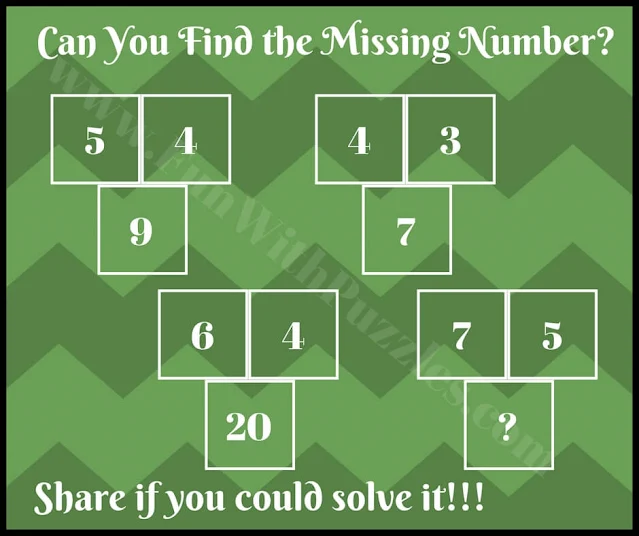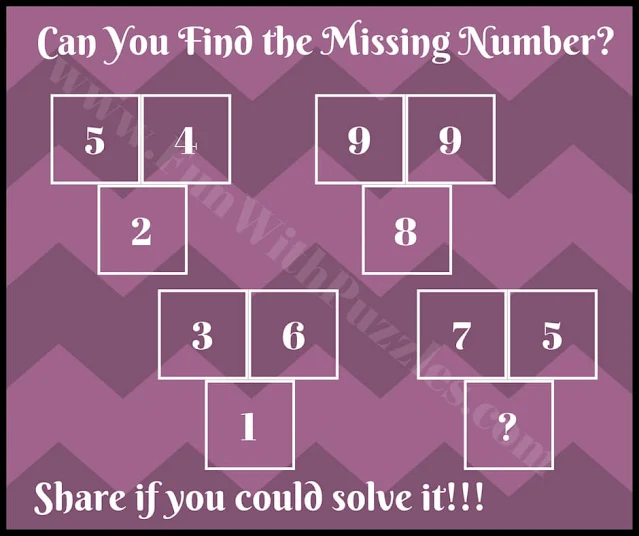I used to love math exercises, especially those related to arithmetic math operations. However, in all these math questions, clear statements were given. Now here are Clever Math Brain Teasers for teen students of Middle School in which there is no statement but only a few numbers are given. One has to find the relationship between these given numbers and then solve it for the missing number.
In these clever Math Brain Teasers, there are four groups of three numbers given. These three numbers have some mathematical pattern or some arithmetic relationship with each other. One has to find this math pattern or relationship among these numbers and then have to solve these math brain teasers for the number which will replace the question mark?1. Easy Picture Math Brain Teaser for Teens2. Fun Picture Math Brain Teaser for Students of Middle School3. Simple Picture Math Number Puzzle Question for Teens4. Tough Picture Math Number Puzzle Question for Teens5. Tricky Picture Math Number Puzzle Riddle for Teens

#### 1 comment:

ಗುಬ್ಬಿ ಮೋಹನ said...

1st 3